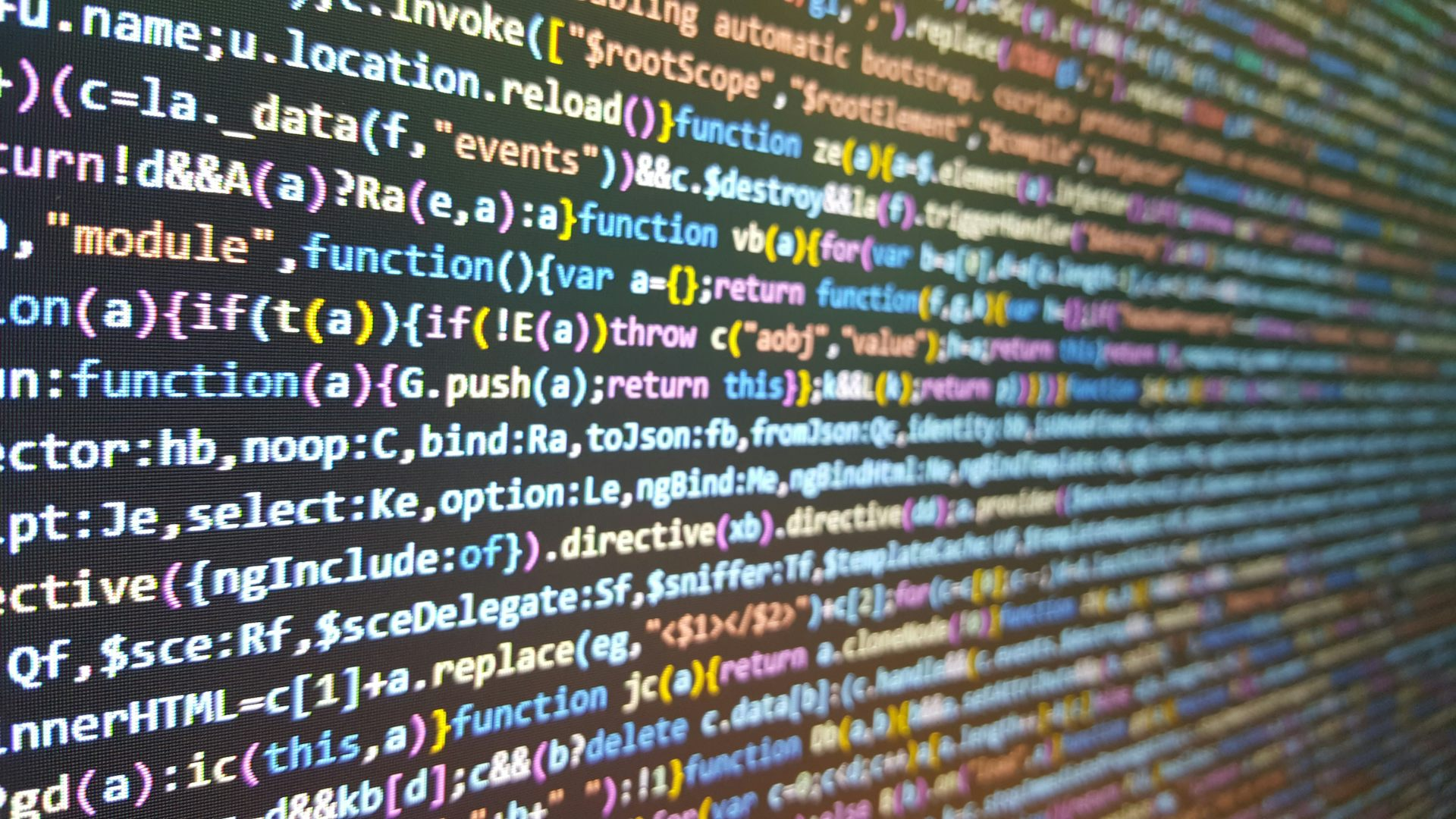# Major quantum computational breakthrough is shaking up physics and maths

MIP* = RE is not a typo. It is a groundbreaking discovery and the catchy title of a recent paper in the field of quantum complexity theory. Complexity theory is a zoo of “complexity classes” – collections of computational problems – of which MIP* and RE are but two.

The 165-page paper shows that these two classes are the same. That may seem like an insignificant detail in an abstract theory without any real-world application. But physicists and mathematicians are flocking to visit the zoo, even though they probably don’t understand it all. Because it turns out the discovery has astonishing consequences for their own disciplines.

In 1936, Alan Turing showed that the Halting Problem – algorithmically deciding whether a computer program halts or loops forever – cannot be solved. Modern computer science was born. Its success made the impression that soon all practical problems would yield to the tremendous power of the computer.

But it soon became apparent that, while some problems can be solved algorithmically, the actual computation will last long after our Sun will have engulfed the computer performing the computation. Figuring out how to solve a problem algorithmically was not enough. It was vital to classify solutions by efficiency. Complexity theory classifies problems according to how hard it is to solve them. The hardness of a problem is measured in terms of how long the computation lasts.

RE stands for problems that can be solved by a computer. It is the zoo. Let’s have a look at some subclasses.

The class P consists of problems which a known algorithm can solve quickly (technically, in polynomial time). For instance, multiplying two numbers belongs to P since long multiplication is an efficient algorithm to solve the problem. The problem of finding the prime factors of a number is not known to be in P; the problem can certainly be solved by a computer but no known algorithm can do so efficiently. A related problem, deciding if a given number is a prime, was in similar limbo until 2004 when an efficient algorithm showed that this problem is in P.

Another complexity class is NP. Imagine a maze. “Is there a way out of this maze?” is a yes/no question. If the answer is yes, then there is a simple way to convince us: simply give us the directions, we’ll follow them, and we’ll find the exit. If the answer is no, however, we’d have to traverse the entire maze without ever finding a way out to be convinced.

Such yes/no problems for which, if the answer is yes, we can efficiently demonstrate that, belong to NP. Any solution to a problem serves to convince us of the answer, and so P is contained in NP. Surprisingly, a million dollar question is whether P=NP. Nobody knows.

## Trust in machines

The classes described so far represent problems faced by a normal computer. But computers are fundamentally changing – quantum computers are being developed. But if a new type of computer comes along and claims to solve one of our problems, how can we trust it is correct?Complexity science helps explain what problems a computer can solve. Phatcharapon/Shutterstock